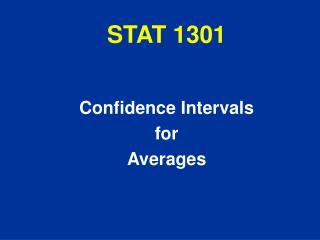Download PresentationSTAT 1301

# STAT 1301

Download Presentation## STAT 1301

- - - - - - - - - - - - - - - - - - - - - - - - - - - E N D - - - - - - - - - - - - - - - - - - - - - - - - - - -
##### Presentation Transcript

1. STAT 1301 Confidence Intervals for Averages

2. Realistic Situation • we usually DON’T KNOWthe population AVG • we take simple random sample of size n • find sample AVG - this is our “best guess” at pop. AVG - “sample statistic” QUESTION: How far is pop. AVG likely to be from sample AVG ?

3. Example: Consumer reporter wants to know average price of unleaded gasoline in Texas on a certain day. • Huge population, can’t get exact average • Take SRS of 100 stations. • Results of sample: AVG(sample) = \$2.15 SD(sample) = \$0.08 • So, we estimate the average price for all stations in Texas to be about \$2.15. How close is this likely to be to the true Texas average ?

4. SE(X) measures this • What’s the problem here? • SE(X) depends on population SD • we don’t know population SD • How could you approximate the population SD? • use sample SD

5. ????? 10 draws with replacement 1, 4, 6, 0, 6, 6, 4, 6, 1, 6

6. Confidence Intervals • Recall -- If population AVG is known, then the chance that a sample AVG will fall within 2 SE’s of the population AVG is about 95% • Turned Around -- If we know the sample AVG, then the population AVG should be within 2 SE’s of this sample AVG about 95% of the time (use “approximate SE”) 95% Confidence Interval for Population AVG X 2 SE(X)

7. Consumer reporter – wants to estimate the average price of regular unleaded gasoline in Texas on a certain day. Sample: 100 stations --- AVG = \$2.15 --- SD =\$0.08 Find a 95% confidence interval for the average for all stations in Texas on that day. Find the corresponding 99.7% CI.

8. Interpetation of 95% Confidence Interval Suppose 100 consumer reporters - each took own sample - each computed a 95% CI IF (for example) the true AVG price is \$1.86 - we could expect about 95 of these 100 CI’s to contain\$ 1.86

9. Note: • For a given sample size n, • if you want higher confidence level, this requires a longer interval

10. General Confidence Intervals X z x SE(X) where z = 1 for 68% CI z = 2 for 95% CI z = 3 for 99.7% CI